# Transfer Function - MCQ Test

## 20 Questions MCQ Test RRB JE for Electrical Engineering | Transfer Function - MCQ Test

Description
This mock test of Transfer Function - MCQ Test for Railways helps you for every Railways entrance exam. This contains 20 Multiple Choice Questions for Railways Transfer Function - MCQ Test (mcq) to study with solutions a complete question bank. The solved questions answers in this Transfer Function - MCQ Test quiz give you a good mix of easy questions and tough questions. Railways students definitely take this Transfer Function - MCQ Test exercise for a better result in the exam. You can find other Transfer Function - MCQ Test extra questions, long questions & short questions for Railways on EduRev as well by searching above.
QUESTION: 1

Solution:
QUESTION: 2

### In the signal flow graph shown in fig. the sum of loop gain of non-touching loops is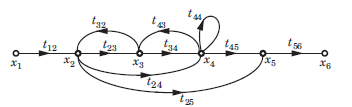Solution:

There cannot be common subscript because subscript refers to node number. If subscript is common, that means that node is in both loop.

QUESTION: 3

### The principles of homogeneity and super position are applied to ____

Solution:

Superposition theorem states that for two signals additivity and homogeneity property must be satisfied and that is applicable for the LTI systems.

QUESTION: 4

The sum of the gains of the feedback paths in the signal flow graph shown in fig.  is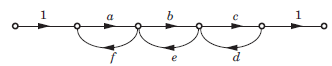Solution:
QUESTION: 5

A control system whose step response is -0.5(1+e-2t) is cascaded to another control block whose impulse response is e-t. What is the transfer function of the cascaded combination?

Solution:

Let h1(t) is the impulse response of system.

h1(t) = d/dt(-0.5(1+e-2t)) h1(t) = e(-2t) h2(t)  = e(-t)

cascading of two system equals to the convolution of both and convolution in time domain is equals to multiplication in frequency domain. so by taking Laplace transform of above two

H1(s) =1/(s+2), H2(s) = 1/(s+1)

hence the transfer function of cascaded system is

H(s) = H1(s)H2(s)

H(s)= 1/(s+1)(s+2)

QUESTION: 6

The overall transfer function C/R of the system shown in fig. will be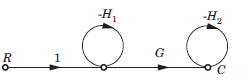Solution: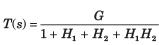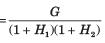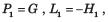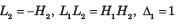QUESTION: 7

Consider the signal flow graphs shown in fig. The transfer 2 is of the graph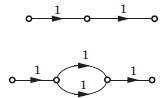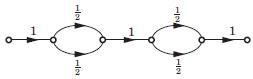Solution:

There are no loop in any graph. So option (B) is correct.QUESTION: 8

Consider the List I and List II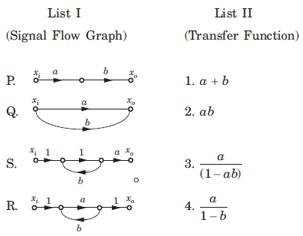The correct match is

Solution:

P. P1 = ab, Δ = 1, L = 0 ,T = ab
Q1 P1 = a, P2 = 6 , Δ = 1, L = Δk = 0,T  = a+b
R. P1 = a, L1 = b, Δ = 1 - b, Δ1 =1,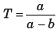S. P1 = a, L1 = ab, Δ = 1 - ab, Δ1 = 1,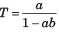QUESTION: 9

For the signal flow graph shown in fig. an equivalent graph is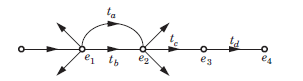Solution:
QUESTION: 10

Consider the block diagram shown in figure.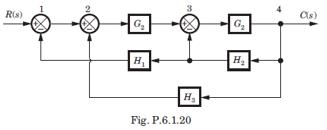For this system the signal flow graph is

Solution:

Option (A) is correct. Best method is to check the signal flow graph. In block diagram there is feedback from 4 to 1 of gain - H1H2 . The signal flow graph of option (A) has feedback from 4 to 1 of gain - H1H2

QUESTION: 11

The block diagram of a system is shown in fig. The closed loop transfer function of this system is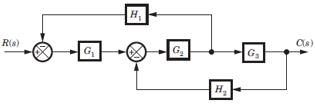Solution:

Consider the block diagram as SFG. There are two feedback loop -G1G2H1 and -G2G3H2 and one forward path G1G2 G3 . So (D) is correct option.

QUESTION: 12

For the system shown in fig. transfer function C(s) R(s) is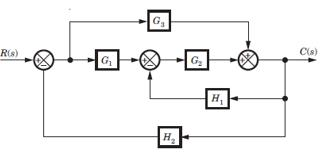Solution:

Consider the block diagram as a SFG. Two forward path G1G2 and G3 and three loops -G1G2 H2, -G2H1, -G3 H2
There are no nontouching loop. So (B) is correct.

QUESTION: 13

In the signal flow graph shown in fig. the transfer function is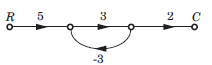Solution:

P1 = 5 x 3 x 2 = 30, Δ = 1 - (3x - 3) = 10
Δ1 = 1,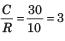QUESTION: 14

In the signal flow graph shown in fig. the gain C/R is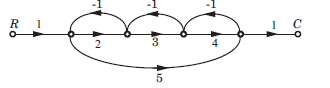Solution:

P1 = 2 x 3 x 4 = 24 , P2 = 1 x 5 x 1 = 5
L1 = -2, L2 = -3, L3 = -4, L4 = -5,
L1L3 = 8, Δ = 1 -(-2 - 3 - 4 - 5) + 8 = 23, Δ1 = 1, Δ2 = 1 - (-3) = 4,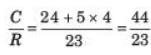QUESTION: 15

The gain C(s)/R(s) of the signal flow graph shown in fig.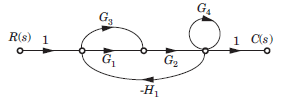Solution:
QUESTION: 16

The transfer function of the system shown in fig. is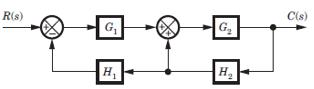Solution:

The correct option is Option C.

L1 = - G1H1         P1 = G1G2

L2 = G2H2           Δ1 = 1 - 0 = 1

Δ = 1 + G1H1 - G1H1

T.f = G1G2 (1) / 1 + G1H1 - G2H2

= G1G2 / 1 + G1H1 - G2H2

QUESTION: 17

For the block diagram shown in fig. transfer function C(s)/R(s) is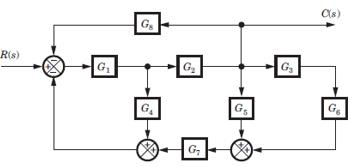Solution:

Four loops -G1G4, -G1G2G5, -G1,G2G5G7 and -G1G2G3G3G7.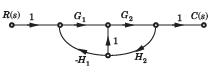There is no nontouching loop. So (B) is correct.

QUESTION: 18

For the block diagram shown in fig. the numerator of transfer function is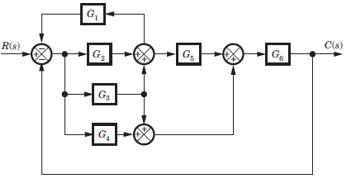Solution:

SFG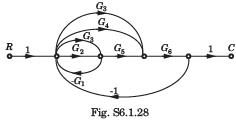P1 = G2G5G6 , P2 = G3G5G6, P3 = G3G6 , P4 = G4G6
If any path is deleted, there would not be any loop.
Hence Δ1 = Δ2 = Δ3 = Δ4 = 1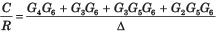QUESTION: 19

For the block diagram shown in fig.  the transfer function C(s)/R(s) is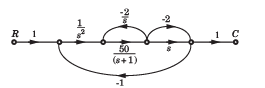Solution: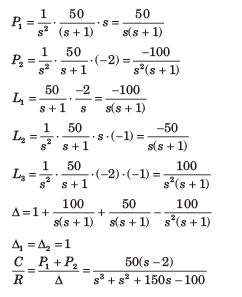QUESTION: 20

In the signal flow graph of figure y/x equals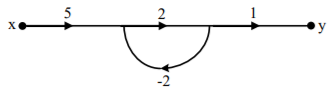Solution:

Transfer function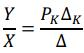PK = 5 x 2 x 1 = 10
ΔK = 1
Δ = 1 - (-4) = 5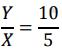= 2